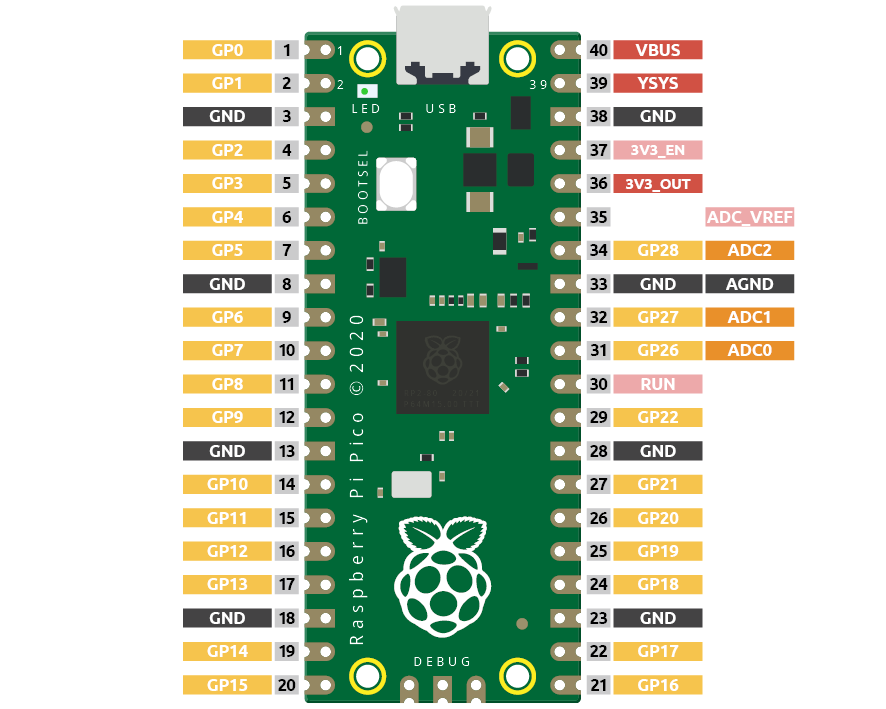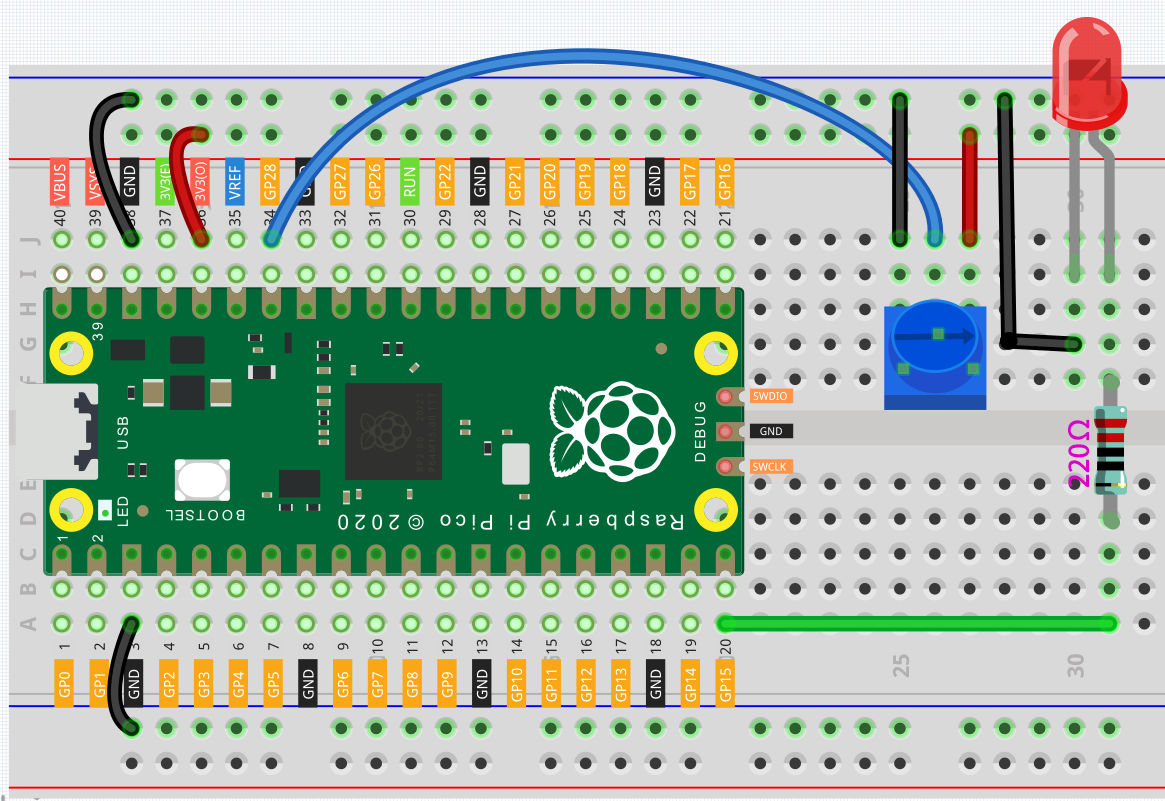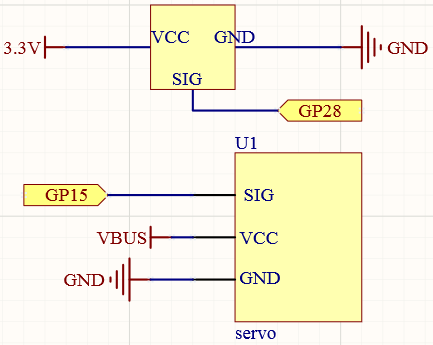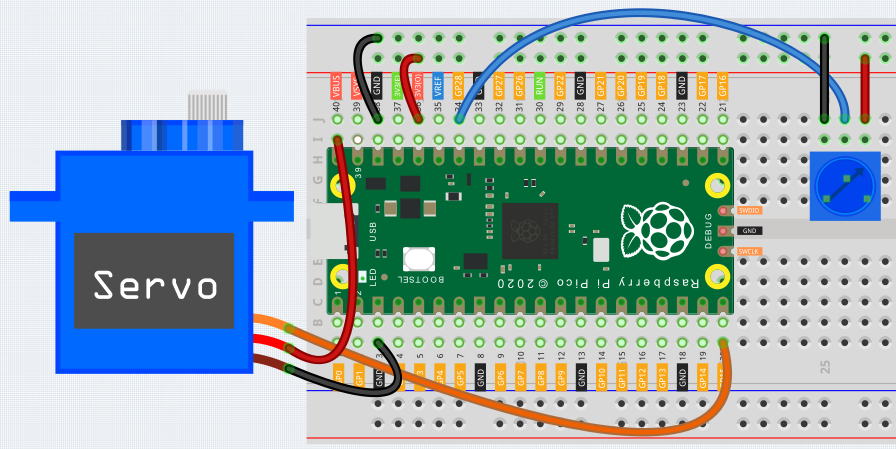# Turn the Knob¶

In the previous projects, we have used the digital input on the Pico. For example, a button can change the pin from low level (off) to high level (on). This is a binary working state.

However, Pico can receive another type of input signal: analog input. It can be in any state from fully closed to fully open, and has a range of possible values. The analog input allows the microcontroller to sense the light intensity, sound intensity, temperature, humidity, etc. of the physical world.Pico has three GPIO pins that can use analog input, GP26, GP27, GP28. That is, analog channels 0, 1, and 2. In addition, there is a fourth analog channel, which is connected to the built-in temperature sensor and will not be introduced here.

In this project, we try to read the analog value of potentiometer.

## Wiring¶1. Connect 3V3 and GND of Pico to the power bus of the breadboard.

2. Insert the potentiometer into the breadboard, its three pins should be in different rows.

3. Use jumper wires to connect the pins on both sides of the potentiometer to the positive and negative power bus respectively.

4. Connect the middle pin of the potentiometer to GP28 with a jumper wire.

5. Connect the anode of the LED to the GP15 pin through a 220Ω resistor, and connect the cathode to the negative power bus.

## Code¶

When the program is running, we can see the analog value currently read by the GP28 pin in the shell. Turn the knob, and the value will change from 0 to 65535. At the same time, the brightness of the LED will increase as the analog value increases.

```import machine
import utime

led = machine.PWM(machine.Pin(15))
led.freq(1000)

while True:
print(value)
led.duty_u16(value)
utime.sleep_ms(200)
```

## What more?¶

Let’s use the potentiometer to swing the servo from left to right!```import machine
import utime

servo = machine.PWM(machine.Pin(15))
servo.freq(50)

def interval_mapping(x, in_min, in_max, out_min, out_max):
return (x - in_min) * (out_max - out_min) / (in_max - in_min) + out_min

def servo_write(pin,angle):
pulse_width=interval_mapping(angle, 0, 180, 0.5,2.5)
duty=int(interval_mapping(pulse_width, 0, 20, 0,65535))
pin.duty_u16(duty)

while True: